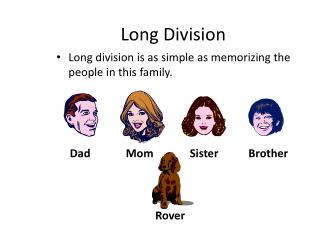DownloadDownload PresentationLong Division

# Long Division

Télécharger la présentation## Long Division

- - - - - - - - - - - - - - - - - - - - - - - - - - - E N D - - - - - - - - - - - - - - - - - - - - - - - - - - -
##### Presentation Transcript

1. Long Division • Long division is as simple as memorizing the people in this family. Dad Mom Sister Brother Rover

2. Long Division • Each person represents a step in the long division process. 3. Subtract 1. Divide Sister 4. Bring down Dad Brother 2. Multiply 5. Repeat or Remainder Mom Rover

3. Step 1 in Long Division 4 1. Divide • Divide 2 into first number in the dividend. 2 ) 9 5 Dad How many 2’s will go into 9? • Think how many 2’s will fit into 9. • Write that number directly above the number you divided into.

4. Step 2 in Long Division 4 2. Multiply • Multiply the divisor times the first number in the quotient. 2 ) 9 5 Mom 8 2 x 4 = 8 • Write your answer directly under the 9 or the number you just divided into.

5. Step 3 in Long Division 4 3. Subtract • Draw a line under the 8. 2 ) 9 5 Sister 8 • Write a subtraction sign next to the 8. 1 • Subtract 8 from 9. • Write your answer directly below the 8.

6. Step 4 in Long Division 4 4. Bring down • Go to the next number in the dividend to the right of the 9. 2 ) 9 5 Brother 8 1 5 • Write an arrow under the 4. • Bring the 4 down next to the 1.

7. Step 5 in Long Division 4 5. Repeat or Remainder • This is where you decide whether you repeat the 5 steps of division. 2 ) 9 5 Rover 8 1 5 • If your divisor can divide into your new number, 15, or if you have numbers in the dividend that have not been brought down, you repeat the 5 steps of division.

8. Step 1 in Long Division 4 7 1. Divide • Divide 2 into your new number, 15. 2 ) 9 5 Dad 8 1 4 • Place your answer directly above the 5 in your quotient.

9. Step 2 in Long Division 4 7 2. Multiply • Multiply your divisor, 2, with your new number in the quotient, 7. 2 ) 9 5 Mom 8 1 5 1 4 • Place your answer directly under the 15.

10. Step 3 in Long Division 4 7 3. Subtract • Draw a line under the bottom 14. 2 ) 9 5 Sister 8 1 5 • Draw a subtraction sign. 1 4 • Subtract & place answer under the line. 1

11. Step 4 in Long Division 4 7 4. Bring down • Look at your dividend to see if there are any more numbers to bring down. 2 ) 9 5 Brother 8 1 5 1 4 • If not, move to step 5. 1

12. Step 5 in Long Division 4 7 R1 5. Repeat or Remainder • Since there are no more numbers to bring down & 2 will not divide into 1, you do not repeat the steps of division. 2 ) 9 5 Rover 8 1 5 1 4 1 • The number left over, 1, becomes the remainder.

13. You’re awesome! You did it! 4 7 R1 2 ) 9 5 8 1 5 Cool Dude! 1 4 You’re so smart! 1 Wolf!